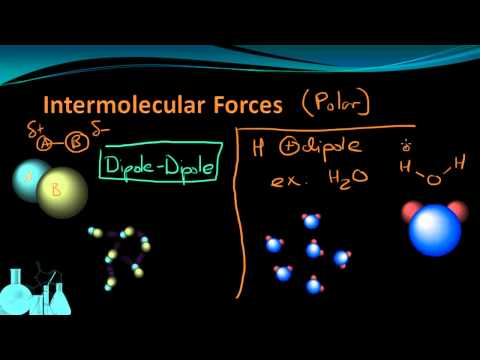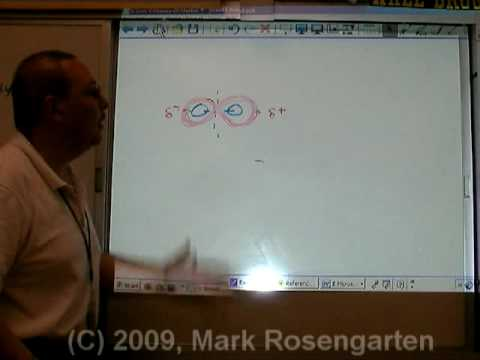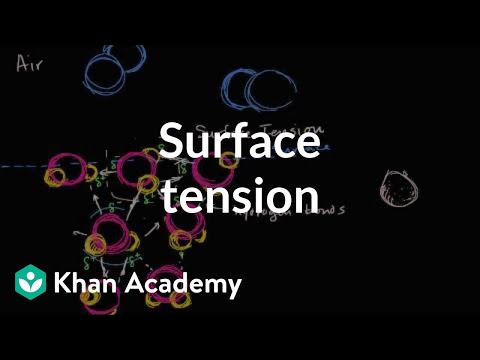Courses

# Test: Intermolecular Forces- States Of Matter

## 13 Questions MCQ Test Chemistry for JEE | Test: Intermolecular Forces- States Of Matter

Description
This mock test of Test: Intermolecular Forces- States Of Matter for JEE helps you for every JEE entrance exam. This contains 13 Multiple Choice Questions for JEE Test: Intermolecular Forces- States Of Matter (mcq) to study with solutions a complete question bank. The solved questions answers in this Test: Intermolecular Forces- States Of Matter quiz give you a good mix of easy questions and tough questions. JEE students definitely take this Test: Intermolecular Forces- States Of Matter exercise for a better result in the exam. You can find other Test: Intermolecular Forces- States Of Matter extra questions, long questions & short questions for JEE on EduRev as well by searching above.
QUESTION: 1

### Q. Which pair of molecules has the strongest dipole-dipole interactions?

Solution:

1. In Case of option (a), We have polar ammonia NH3 but non polar CH4, tetrahedral and all forces balanced, so weak attraction between the two.

2. Option (B) is correct.
NH3 is a fairly polar molecules due to the strong electronegativity of N and its pyrimidal shape with a lone pair of electrons at the peak. So two of the molecules have a strong attraction for one another.

3. In Case of option (c), both molecules being non polar due to symetrical structure in tetrahedrons .

4. In Case of option (d), both molecules are non polar, structure is O=C=O

QUESTION: 2

### Intermolecular forces can be out of the following:

Solution:

Intermolecular force can only be Vaan der Waals force acting between two molecules.

QUESTION: 3

### Based on the following statements I and IS, select the correct answer from the codes given. Statement I : Three states of matter are the result of balance between intermolecular forces and thermal energy of the molecules. Statement II : Intermolecular forces tend to keep the molecules together but thermal energy of molecules tends to keep them apart.

Solution:

Thermal energy is the energy of a body arising from motion of its atoms or molecules. It is directly proportional to the temperature of the substance. It is the measure of average kinetic energy of the particles of the matter and is thus responsible for movement of particles. This movement of particles is called thermal motion. We have already learnt that intermolecular forces tend to keep the molecules together but thermal energy of the molecules tends to keep them apart. Three states of matter are the result of balance between intermolecular forces and the thermal energy of the molecules.

QUESTION: 4

In comparing gases with liquids , gases have ........ compressibility and...........density.

Solution:

In a gas, the distance between molecules, whether monatomic or polyatomic, is very large compared with the size of the molecules; thus gases have a low density and are highly compressible.

Density: The molecules of a liquid are packed relatively close together. Consequently, liquids are much denser than gases.

QUESTION: 5

Van der waals forces include the following except

Solution:

Van der Waals forces are distance-dependent forces between atoms and molecule not associated with covalent or ionic chemical bonds. Sometimes the term is used to encompass all intermolecular forces, although some scientists only include London dispersion force, Debye force, and Keeson force.

QUESTION: 6

Dipole-dipole interaction energy between stationary polar molecules is proportional to x and that between rotating molecules is proportional to y. Assume distance between polar molecules as r, then x and y are

Solution:

Dipole-dipole interaction energy between stationary polar molecules is proportional to 1/ r3 and that between rotating polar molecules is proportional to 1/ r6 where ‘r’ is the distance between polar molecules

Besides dipole - dipole interaction, polar molecules can interact by London forces also.

*Multiple options can be correct
QUESTION: 7

Direction (Q. Nos. 7-10) This section contains 4 multiple choice questions. Each question has four choices (a), (b), (c) and (d), out of which ONE or  MORE THANT ONE  is correct.

Q. Select the correct alternate(s).

Solution:

All of the above are the properties of gases.

QUESTION: 8

The interaction energy of London force is inversely proportional to sixth power of the distance between two interacting particles but their magnitude depends upon

Solution:

London dispersion forces operate only over very short distance. The energy of interaction varies as
1/(distance between two interacting particles)

Large or more complex the molecules, greater is the magnitude of London forces.

This is obviously due to the fact that the large electron clouds are easily distorted or polarised.
Hence, greater the polarisability of the interacting particles, greater is the magnitude of the interaction energy.

QUESTION: 9

Hydrogen bonding reduces the quality of water molecules to

Solution:

The formation of hydrogen bonds is an important quality of the water molecules make hydrogen bonds with each other, water takes on some unique chemical characteristics compared to other liquids and, since living things have a high water content, understanding these chemical features is key to understanding life. In liquid water, hydrogen bonds are constantly formed and broken as the water molecules slide past each other.

QUESTION: 10

Atom which must be present in hydrogen bonding is

Solution:

A hydrogen bond is a partially electrostatic attraction b/w a hydrogen atom which is bound to a more electronegative atom such as N, O and F etc. And also hydrogen bonding occurs only b/w molecules in which H atom bounded to a electronegative atom.

QUESTION: 11

Direction (Q. Nos. 11-13) This section contains a paragraph, wach describing theory, experiments, data etc. three Questions related to paragraph have been given.Each question have only one correct answer among the four given options (a),(b),(c),(d)

Dipole-dipole forces act between the molecules possessing permanent dipole. Ends of dipoles possess ‘partial charges’. The partial charge is

Solution:

Partial charge is a small charge developed by displacement of electrons. It is less than unit electronic charge and is represented as δor δ–

QUESTION: 12

Stronger intermolecular forces result in higher boiling point. Strength of London forces increases with number of electrons in the molecule. Boiling point of

I. HF 293 K
II. HCl 189 K
III. HBr 206 K
IV. HI 238 K

Q.
Based on the boiling points, predominant force which gives variation of boiling point of HCI < HBr < HI is

Solution:

HF - Hydrogen bonding HCl,HBr,HI→ Dipole-dipole interaction, London-dispersion force.

QUESTION: 13

Stronger intermolecular forces result in higher boiling point. Strength of London forces increases with number of electrons in the molecule. Boiling point of

I. HF 293 K
II. HCl 189 K
III. HBr 206 K
IV. HI 238 K

Q.
HF has highest boiling point because of

Solution:

It is because of hydrogen bonding . Since F is the most electronegative element , its hydrogen bonding tendency is strongest which makes boiling point of HF increase.

Track your progress, build streaks, highlight & save important lessons and more!

### Similar Content### Related tests